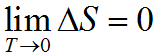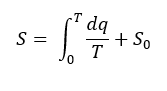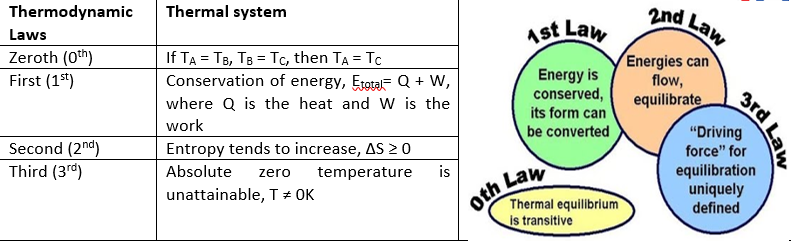# Third law of thermodynamics

Chemical Thermodynamics: Third law of thermodynamics

This law is a statistical law of nature. It is associated with entropy and the impossibility of reaching absolute zero of temperature. For substances undergoing an isothermal process, the entropy change goes to zero as T (in K) goes to zero as they reach equilibrium.The law is applicable for pure substances and mixtures. On the Kelvin Temperature Scale, T = 0 K is often referred to as the Absolute Zero Point. Likewise, there does not exist any finite sequence of a cyclical process, which can cool a body to zero Kelvin (absolute zero). Temperatures as low as 2.0 x 10-8 K can be achieved at laboratory scale, but absolute zero will remain ever elusive. This means that there is simply nowhere to “put” that last little bit of energy.

For a closed system in thermodynamic equilibrium, the Ssys approaches a constant value as the T goes to 0. If there is a unique ground state with minimum energy at zero Kelvin, then the entropy at zero Kelvin is ZERO. However, if there is a degeneracy with respect to the number of micro states at absolute zero, then there will be some residual entropy. Theoretically, at 0K all modes of motions such as vibration, rotation and translation come to a halt.

The entropy of a pure crystalline substance at absolute zero is 0 and they make the most orderly system

S = k lnW = k ln 1 = 0

Where K is the Boltzmann’s constant and W represents the number of microstates of a specific system.Figure 1: Entropy of a pure crystalline substance

The most important application of the third law of thermodynamics is that it aids in the calculation of absolute entropy of a substance at any temperature T.

S=2.303CplogT

Where Cpis the heat capacity of the substance at constant pressure which should remain constant from temperature 0 to T.The calculation of absolute entropy, S is important because it is related to other state and path functions such as

Helmholtz free energy, F: F = U – TS, where U is the internal energy

Gibbs free energy, G: G = H – TS, where H is the enthalpy

Limitations of the law

• Glassy solids at 0oK have entropy greater than zero.
• Solids with mixtures of isotopes do not have zero entropy at 0oK. The entropy of solid chlorine is not zero at 0oK.
• Crystals ofN2O, NO, H2O, CO etc. do not have perfect order even if they are at 0oK, which means that their entropy is not equal to zero.Figure 2: Entropy of crystals as temperature increases when the substance undergoes motion in translational, rotational and vibrational mode.

Table 1: Summary of thermodynamic laws: